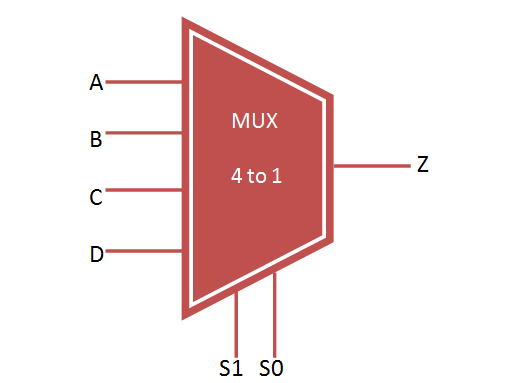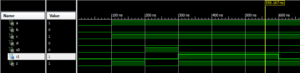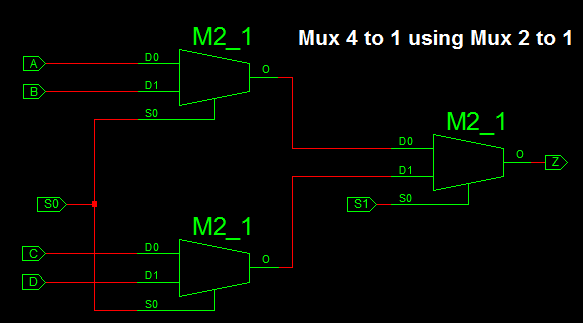# VHDL 4 to 1 Mux (Multiplexer)

### Multiplexer

Multiplexer (MUX) select one input from the multiple inputs and forwarded to output line through selection line. It consist of 2 power n input and 1 output. The input data lines are controlled by n selection lines.

For Example, if n = 2 then the mux will be of 4 to 1 mux with 4 input, 2 selection line and 1 output as shown below.### Truth Table for Multiplexer 4 to 1### Mux 4 to 1 design using Logic Gates### VHDL Code For 4 to 1 Multiplexer

```library IEEE;
use IEEE.STD_LOGIC_1164.all;

entity mux_4to1 is
port(

A,B,C,D : in STD_LOGIC;
S0,S1: in STD_LOGIC;
Z: out STD_LOGIC
);
end mux_4to1;

architecture bhv of mux_4to1 is
begin
process (A,B,C,D,S0,S1) is
begin
if (S0 ='0' and S1 = '0') then
Z <= A;
elsif (S0 ='1' and S1 = '0') then
Z <= B;
elsif (S0 ='0' and S1 = '1') then
Z <= C;
else
Z <= D;
end if;

end process;
end bhv;
```

### VHDL TestBench Code for 4 to 1 Multiplexer

```LIBRARY ieee;
USE ieee.std_logic_1164.ALL;

ENTITY tb_mux IS
END tb_mux;

ARCHITECTURE behavior OF tb_mux IS

– Component Declaration for the Unit Under Test (UUT)

COMPONENT mux_4to1
PORT(
A : IN  std_logic;
B : IN  std_logic;
C : IN  std_logic;
D : IN  std_logic;
S0 : IN  std_logic;
S1 : IN  std_logic;
Z : OUT  std_logic
);
END COMPONENT;

– Inputs
signal A : std_logic := '0';
signal B : std_logic := '0';
signal C : std_logic := '0';
signal D : std_logic := '0';
signal S0 : std_logic := '0';
signal S1 : std_logic := '0';

--Outputs
signal Z : std_logic;

BEGIN

– Instantiate the Unit Under Test (UUT)
uut: mux_4to1 PORT MAP (
A => A,
B => B,
C => C,
D => D,
S0 => S0,
S1 => S1,
Z => Z
);

– Stimulus process
stim_proc: process
begin
– hold reset state for 100 ns.
wait for 100 ns;

A <= '1';
B <= '0';
C <= '1';
D <= '0';

S0 <= '0'; S1 <= '0';

wait for 100 ns;

S0 <= '1'; S1 <= '0';

wait for 100 ns;

S0 <= '0'; S1 <= '1';

wait for 100 ns;

S0 <= '0'; S1 <= '1';

wait for 100 ns;

end process;

END;
```

### Output Waveform for 4 to 1 MultiplexerAnother Method of Constructing VHDL 4 to 1 mux is by using 2 to 1 Mux. For that implementation first we have write VHDL Code for 2 to 1 Mux and Port map 3 times 2 to 1 mux to construct VHDL 4 to 1 Mux.

### 4 to 1 Mux Implementation using 2 to 1 Mux### VHDL Code for 2 to 1 Mux

```
library IEEE;
use IEEE.STD_LOGIC_1164.ALL;

entity mux2_1 is
port(A,B : in STD_LOGIC;
S: in STD_LOGIC;
Z: out STD_LOGIC);
end mux2_1;

architecture Behavioral of mux2_1 is

begin

process (A,B,S) is
begin
if (S ='0') then
Z <= A;
else
Z <= B;
end if;
end process;

end Behavioral;

```

### VHDL 4 to 1 Mux using 2 to 1 Mux

```
library IEEE;
use IEEE.STD_LOGIC_1164.ALL;

entity mux4_1 is
port(

A,B,C,D : in STD_LOGIC;
S0,S1: in STD_LOGIC;
Z: out STD_LOGIC
);
end mux4_1;

architecture Behavioral of mux4_1 is
component mux2_1
port( A,B : in STD_LOGIC;
S: in STD_LOGIC;
Z: out STD_LOGIC);
end component;
signal temp1, temp2: std_logic;

begin
m1: mux2_1 port map(A,B,S0,temp1);
m2: mux2_1 port map(C,D,S0,temp2);
m3: mux2_1 port map(temp1,temp2,S1,Z);

end Behavioral;

```

VHDL Testbench and Simulation Waveform for 4 to 1 mux using 2 to 1 mux is same as the above implementation.

### 1 thought on “VHDL 4 to 1 Mux (Multiplexer)”

1.very good explanation, thank you!